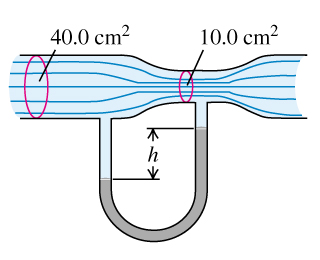Problem: The horizontal pipe, shown in the figure, has a cross-sectional area of 40.0 cm2 at the wider portions and 10.0 cm2 at the constriction. Water is flowing in the pipe, and the discharge from the pipe is 6.00 10 - 3 m3/s (6.00 L/s). The density of mercury is ρHg = 13.6 103 kg/m3 and the density of water is ρw = 1.00 103 kg/m3.a) Find the flow speed at the wide portion.b) Find the flow speed at the narrow portion.c) What is the pressure difference between these portions?d) What is the difference in height between the mercury columns in the U-shaped tube?

Problem Details

The horizontal pipe, shown in the figure, has a cross-sectional area of 40.0 cm2 at the wider portions and 10.0 cm2 at the constriction. Water is flowing in the pipe, and the discharge from the pipe is 6.00 10 - 3 m3/s (6.00 L/s). The density of mercury is ρHg = 13.6 103 kg/m3 and the density of water is ρw = 1.00 103 kg/m3.
a) Find the flow speed at the wide portion.
b) Find the flow speed at the narrow portion.
c) What is the pressure difference between these portions?
d) What is the difference in height between the mercury columns in the U-shaped tube?